# Periodic Planar Collisionless Three Body Orbits With Unequal Mass

The 1349 periodic orbits of planar three-body problem with unequal mass and zero angular momentum

Originator: X. Li, Y. Jing, and S. Liao

The three-body problem seeks solutions for the orbits of three masses under the mutual influence of gravity. Periodic solutions are very hard to find.

(1349 elements)

## Examples

### Basic Examples

Extract velocities:

 In:=Out=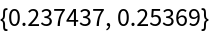Maximum value of period:

 In:=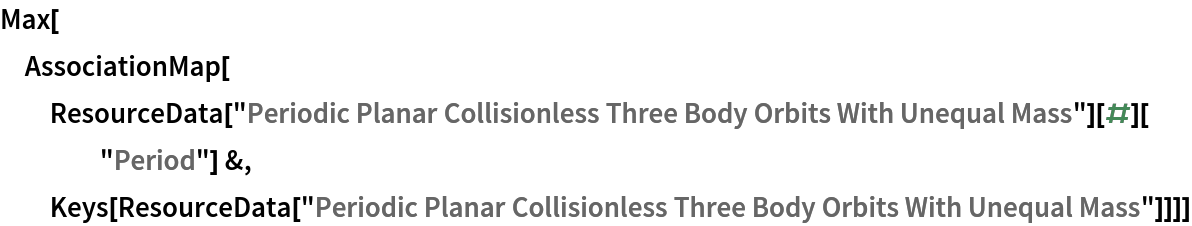Out=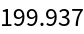All initial velocities:

 In:=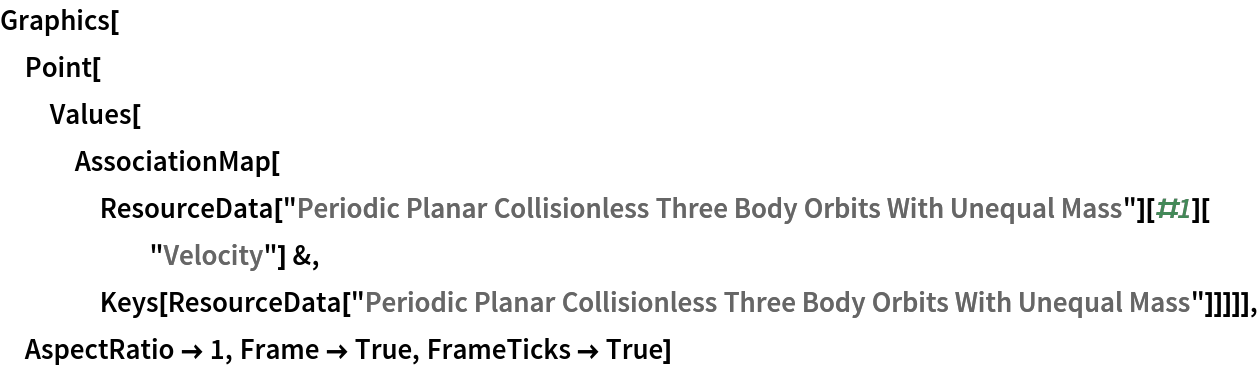Out=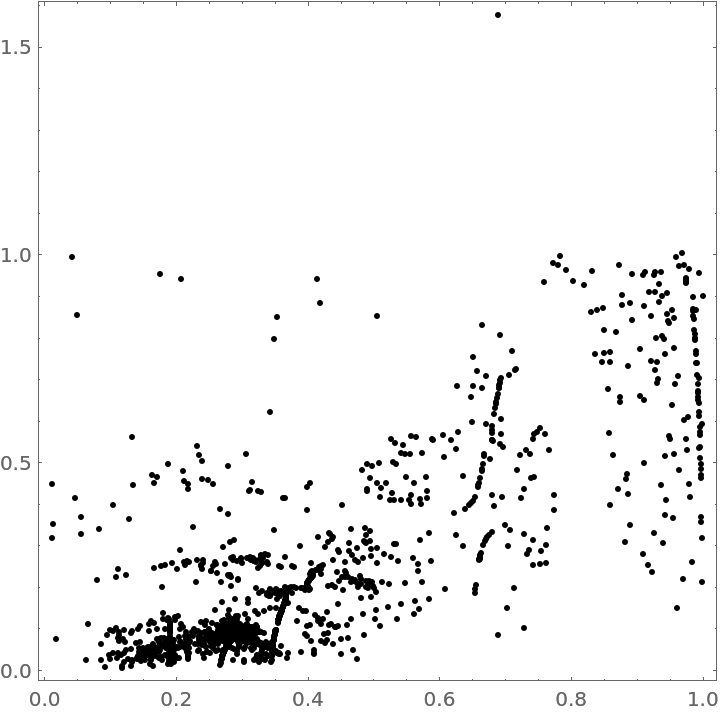Use NBodySimulation to solve an orbit:

 In:=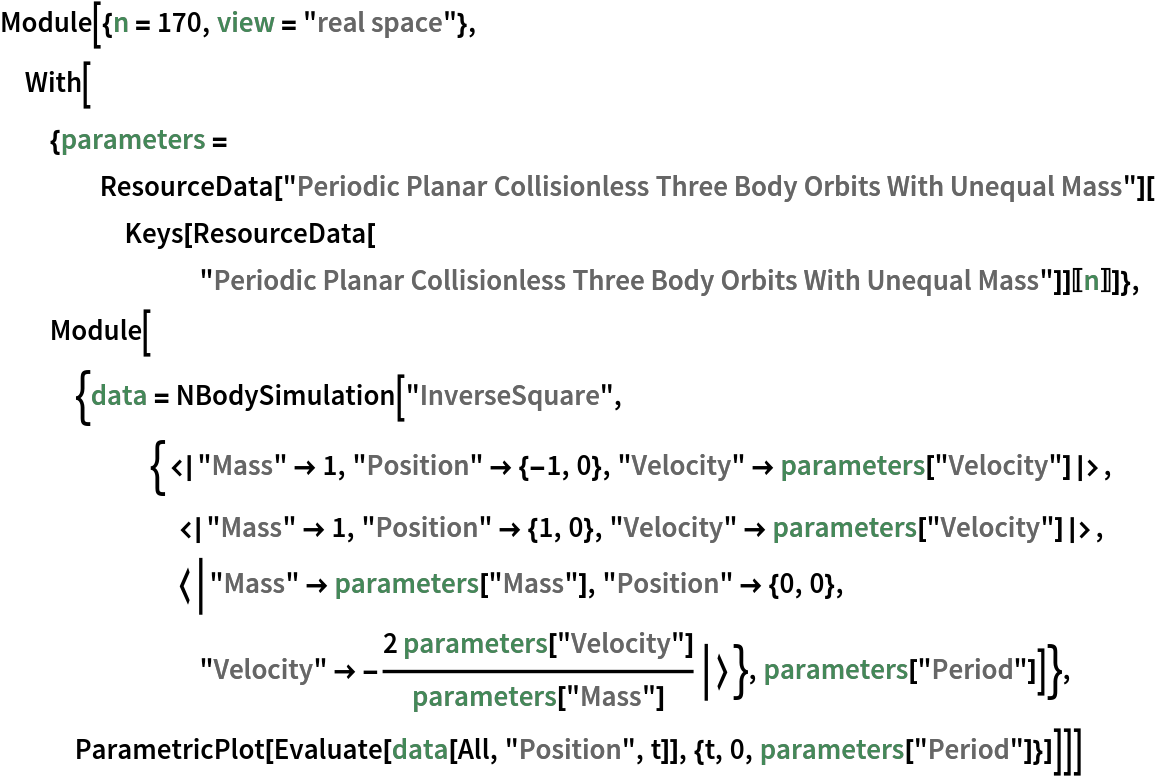Out=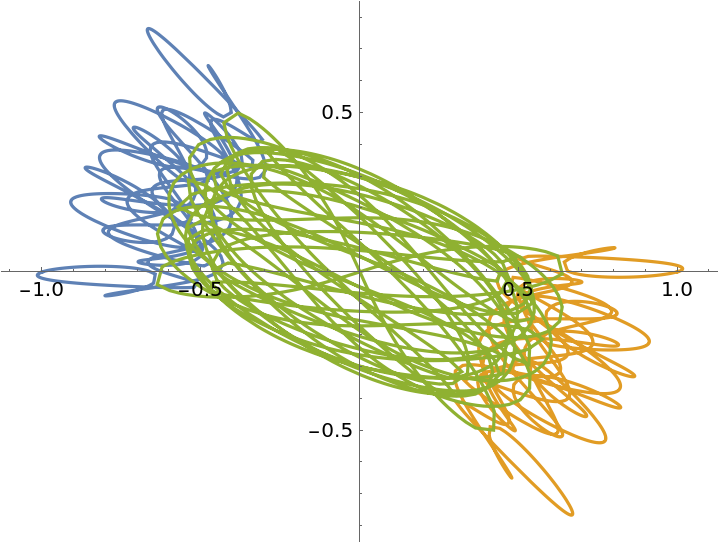Plot in a "shape sphere" using Jacobi three-body coordinates:

 In:=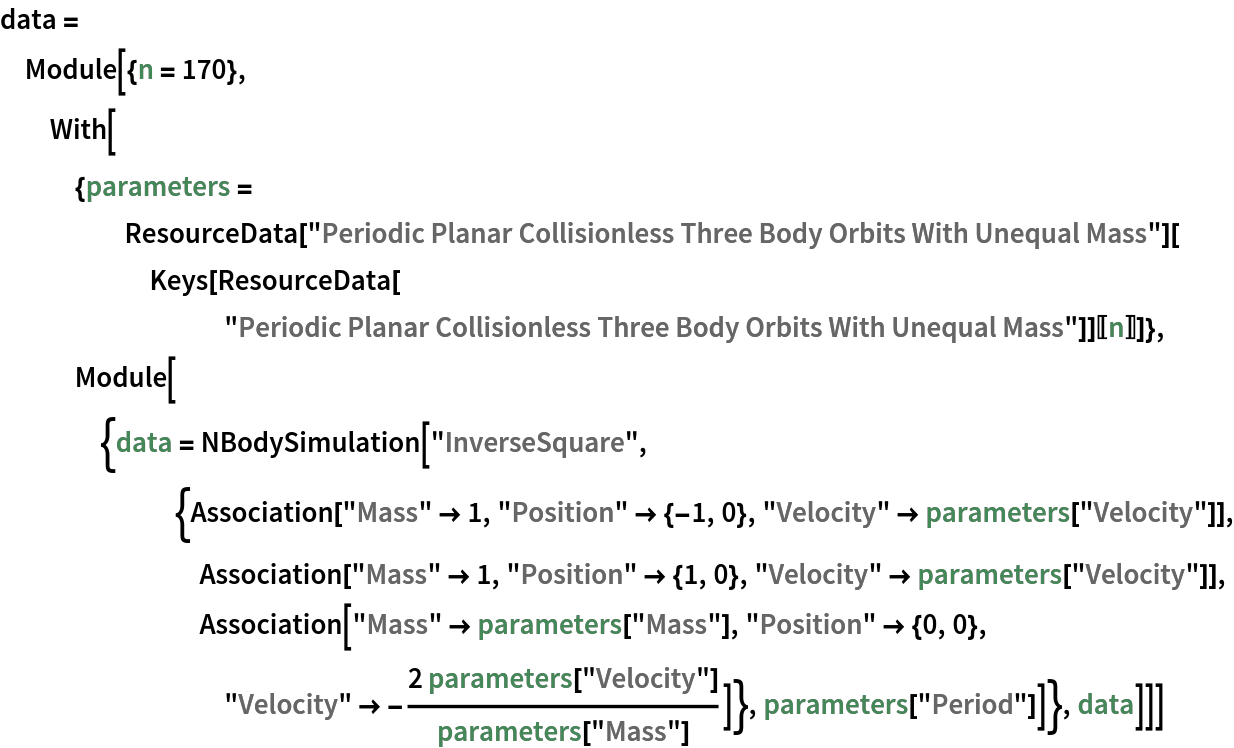Out=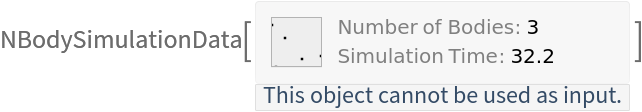In:=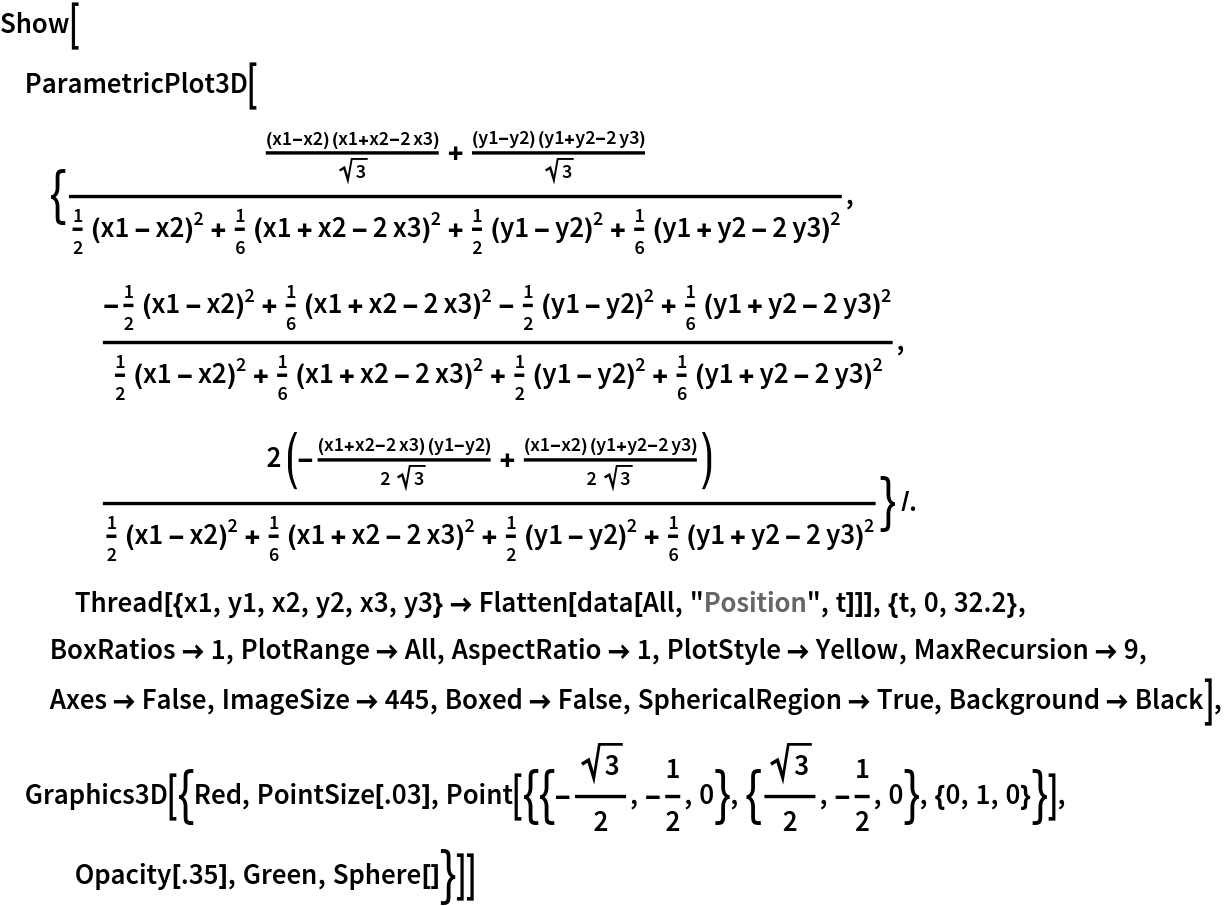Out=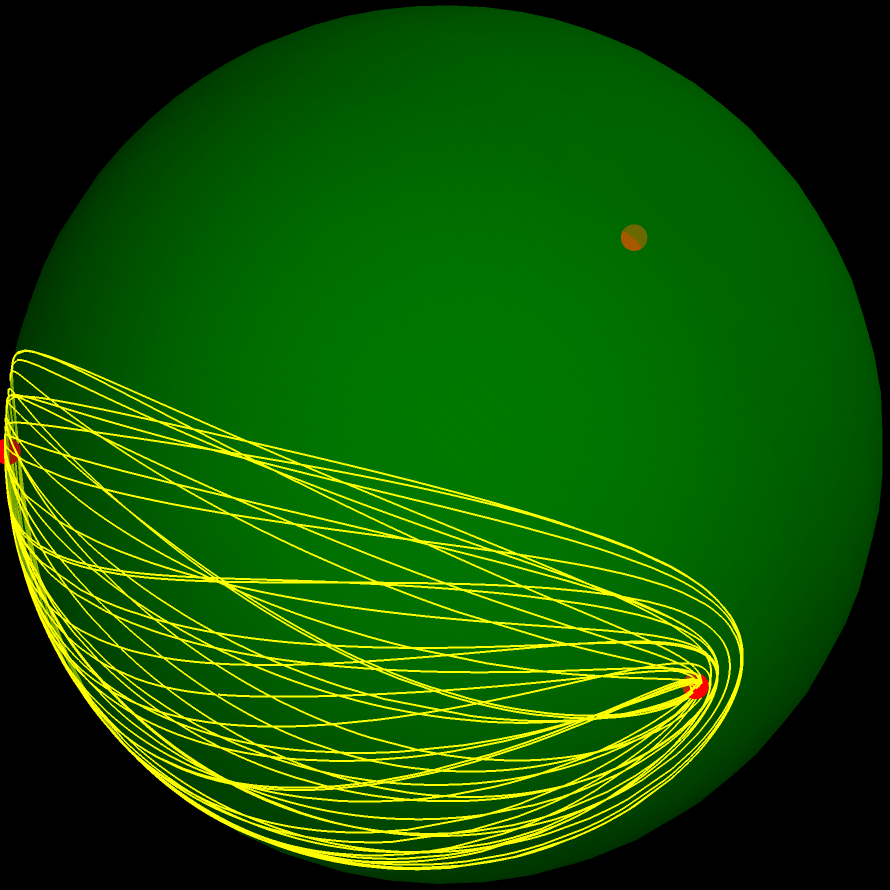Plot orbits from different families:

 In:=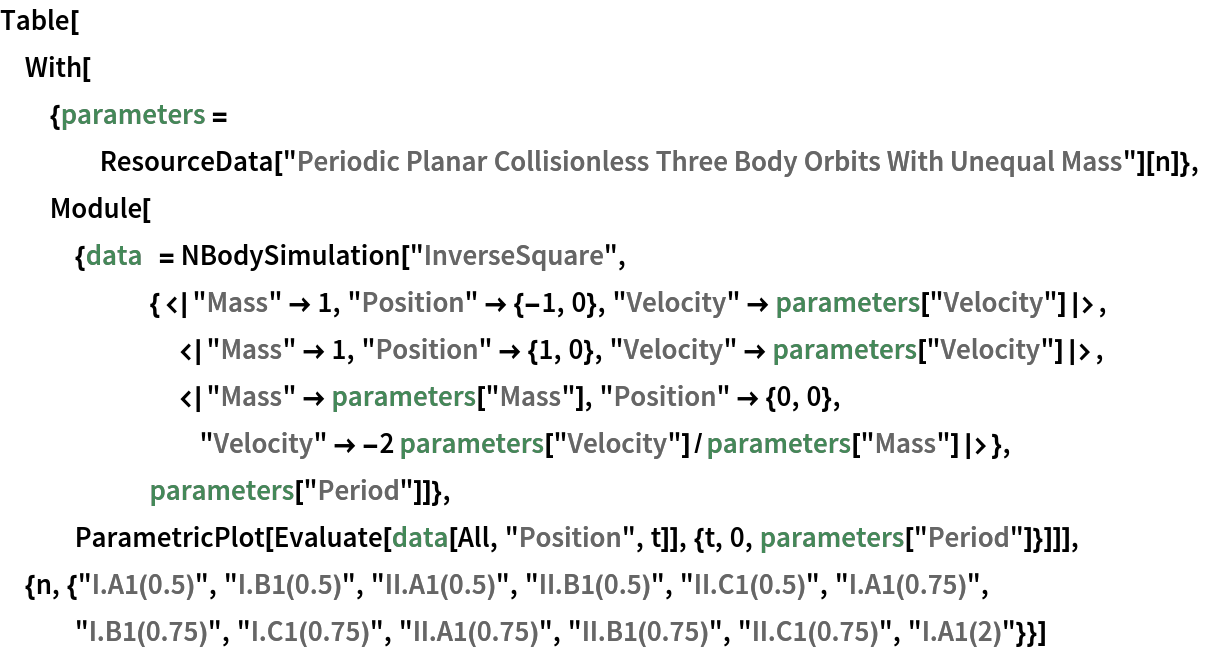Out=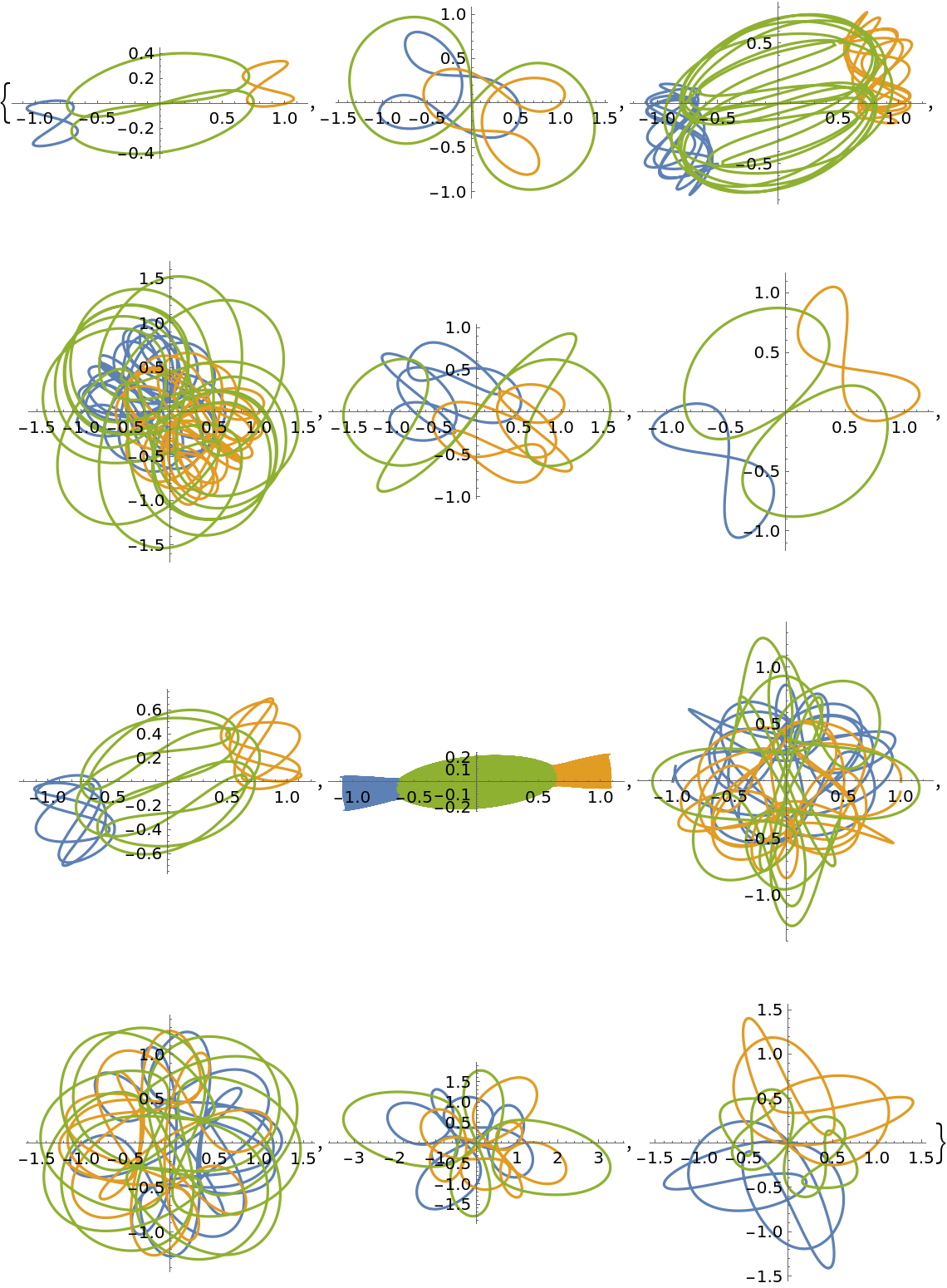Enrique Zeleny, "Periodic Planar Collisionless Three Body Orbits With Unequal Mass" from the Wolfram Data Repository (2021)